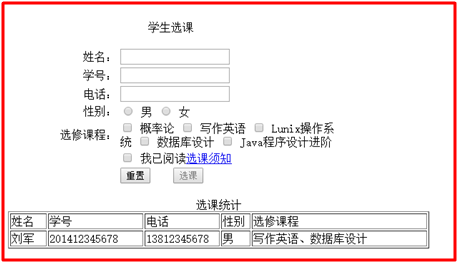2018-12-02 03:50

# 利用Dreamweaver制作如下表单和表格标题行（图中表格第二行不制作，表格标题行可以用代码生成）1、 用户名长度由6至12位字母与数字组成，以字母开头，字母区分大小写，用正则表达式验证。
2、 学号以“20”开头，共12位数字，用正则表达式验证。
3、 电话是11位手机号，用正则表达式验证。
4、 必须选中“我已阅读选课须知”后，“选课”按钮才可以使用。
5、 “选课须知”设置为超链接，点击该超链接时使用window.open( )函数打开一个新窗口，在其中显示“选课须知”页面内容（“选课须知”页面须自制作，内容自定，不少于500字），选课须知页面打开之后，设定15秒之后自动关闭窗口。
6、 用户输入选课全部信息后（选修课至少选2门），点击“选课”按钮，象示例行那样在表格下方插入新的一行，插入的行不允许有相同的学号。

• 写回答
• 好问题 提建议
• 关注问题
• 收藏
• 邀请回答

#### 1条回答默认 最新

•可爱的小书包 2018-12-02 06:39
已采纳
``````<!DOCTYPE html>
<!--suppress JSUnresolvedFunction -->
<html lang="cn">

<meta charset="UTF-8">
<title>学生选课</title>
<style>
.choose_course {
width: 400px;
margin: 0 auto;
}
.show_course {
margin-top: 50px;
}
h3 {
margin: 5px auto;
text-align: center;
}
form, table {
margin: 0 auto;
}
tr td:first-child {
width: 90px;
text-align: right;
}
tr :last-child {
text-align: left;
}
tr td input[type='text'] {
width: 200px;
}
.error {
color: #f00;
}
.success {
color: #00ff00;
}
.show_course table {
border: 1px solid #000000;
}
.show_course table td {
text-align: center;
border: 1px solid #000000;
}
</style>

<body>
<div class="choose_course">
<h3>学生选课</h3>
<form action="#" method="post">
<table>
<tr>
<td style="vertical-align: top">姓名：</td>
<td>
<input type="text" name="name"/>
</td>
</tr>
<tr>
<td style="vertical-align: top">用户名：</td>
<td>
<span class="error" hidden><br>用户名长度只能是6至12位字母与数字</span>
<span class="error" hidden><br>用户名只能以英文字母开头</span>
</td>
</tr>
<tr>
<td style="vertical-align: top">学号：</td>
<td>
<input type="text" name="id"/>
<span class="error" hidden><br>学号必须以20开头</span>
<span class="error" hidden><br>学号长度为必须12位</span>
<span class="error" hidden><br>学号已被占用</span>
<span class="success" hidden><br>学号可用</span>
</td>
</tr>
<tr>
<td style="vertical-align: top">电话：</td>
<td>
<input type="text" name="phone"/>
<span class="error" hidden><br>手机号码为11位</span>
</td>
</tr>
<tr>
<td>性别：</td>
<td>
</td>
</tr>
<tr>
<td style="vertical-align: top">选修课程：</td>
<td>
<input type="checkbox" name="course" value="概率论"/>概率论
<input type="checkbox" name="course" value="写作英语"/>写作英语
<input type="checkbox" name="course" value="Linux操作系统"/>Linux操作系统
<input type="checkbox" name="course" value="数据库设计"/>数据库设计
<input type="checkbox" name="course" value="Java程序设计进阶"/>Java程序设计进阶
<span class="error" hidden><br>课程必须勾选两门及以上</span>
</td>
</tr>
<tr>
<td></td>
<td>
</td>
</tr>
<tr>
<td>
</td>
<td>
<input type="reset" value="重置"/>
<input type="submit" value="选课" disabled="disabled"/>
</td>
</tr>
</table>
</form>
</div>
<div class="show_course">
<h3>选课统计</h3>
<table>
<tr>
<td>姓名</td>
<td>学号</td>
<td>电话</td>
<td>性别</td>
<td>选修课程</td>
</tr>
<tr>
<td class="name">刘军</td>
<td class="id">201412345678</td>
<td class="phone">12345678901</td>
<td class="sex">男</td>
<td class="courses">写作英语、数据库设计</td>
</tr>
</table>
</div>
</body>
<script src="https://cdn.bootcss.com/jquery/1.12.1/jquery.js"></script>
<!--检查表单-->
<script>
\$(function () {
//检查用户名
\$(this).next(".error").show().siblings("span").hide();
return;
}
let re = /[a-z]\w{5,11}/i;
if (!re.test(\$(this).val())) {
\$(this).siblings(".error").eq(1).show().siblings("span").eq(0).hide();
return;
}
\$(this).siblings(".error").hide();
});
//检查学号
\$("input[name='id']").blur(function () {
let idNum = \$(this).val();
if (!idNum.startsWith(20)) {
\$(this).siblings("span").eq(0).show().siblings("span").hide();
return;
}
if (idNum.length != 12) {
\$(this).siblings("span").eq(1).show().siblings("span").hide();
return;
}
let idArr = [];
\$(".show_course table .id").each(function (index, item) {
idArr.push(\$(this).text());
});
if (idArr.includes(idNum)) {
\$(this).siblings("span").eq(2).show().siblings("span").hide();
return;
}
\$(this).siblings("span").eq(3).show().siblings("span").hide();
});
//检查电话号码
\$("input[name='phone']").blur(function () {
let re = /\d{11}/;
if (!re.test(\$(this).val())) {
\$(this).siblings("span").eq(0).show().siblings("span").hide();
return;
}
\$(this).siblings("span").hide();
});

//检查选修课程是否满足
\$("input[name='course']").click(function(){
if (\$(this).siblings(":checked").length < 1) {
\$(this).siblings("span").show();
return;
}
\$(this).siblings("span").hide();
});
//检查当前页面错误的信息条数
let errorLength = \$(".error:visible").length;
if (errorLength !== 0) {
return;
}
//检查当前页面正确的信息条数
let successLength = \$(".success:visible").length;
if (successLength !== 1) {
return;
}
//检查性别是否为空
if (\$("input[name='sex']:checked").val() === undefined) {
return;
}
//检查选修课程是否满足
if (\$("input[name='course']:checked").val() === undefined) {
return;
}
\$("input[type='submit']").prop("disabled", !\$(this).prop("checked"));
});
})
</script>
<!--添加表单-->
<script>
\$("form").submit(function(){
let name = \$("input[name='name']").val();
let id = \$("input[name='id']").val();
let phone = \$("input[name='phone']").val();
let sex = \$("input[name='sex']").val();
let courseArr = [];
\$("input[name='course']:checked").each(function () {
courseArr.push(\$(this).val())
});
let newCol = `
<tr>
<td class="name">` + name + `</td>
<td class="id">` + id + `</td>
<td class="phone">` + phone + `</td>
<td class="sex">` + sex + `</td>
<td class="courses">` + courseArr.join("、") + `</td>
</tr>
`;
\$(newCol).appendTo(".show_course table");
//清空所有的输入
\$("input[name]").val("").prop("checked",false).siblings(".success").hide();
//阻止form表单提交
return false;
});
</script>
<!--设置超链接-->
<script>
\$("a").click(function () {
myWindow = window.open('', '', 'width=200,height=100');
myWindow.document.write("<p>这是选课须知</p>");
myWindow.focus();
});
</script>
</html>

``````
已采纳该答案
评论
解决 无用
打赏 举报